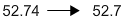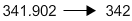# Rounding Decimals

Print
Rate 0 stars
Common Core
• Lesson size:
Message preview:
Someone you know has shared lesson with you:

To play this lesson, click on the link below:

https://www.turtlediary.com/lesson/rounding-decimals.html

Hope you have a good experience with this site and recommend to your friends too.

Login to rate activities and track progress.
Login to rate activities and track progress.

Rounding off a decimal is used to find approximate values of decimals.

Similarly, you probably don't need to say that your house is 2.015 miles from the grocery store. Instead, you might say that the store is about 2 miles away.

Like addition and subtraction, rounding decimals is very similar to rounding whole numbers.

Here's what you need to do, in three simple steps:

• Figure out what place you need to round to - circle that digit if it helps you.
• Look at the digit right after the one you want to round to.
• If this digit is 5 or bigger, round up (add 1 to the digit you are rounding to - this is the number you circled!).
• If it's smaller than 5, round down (leave the digit you are rounding to as it is).
• Get rid of all of the numbers to the right of the digit you are rounding to.

Let's take a look at some examples.

## Example 1

Round 52.74 to the nearest tenth.

 Tens Ones . Tenths Hundredths 5 2 . 7 4

The tenth is the first digit after the decimal point, just to the right of the ones (52.74).

Let's look at the digit right after it. The digit to the right of 7 is 4.

52.74

4 is smaller than 5, so we leave our tenths digit the same (as 7 only).

52.74

Finally, we get rid of the numbers to the right of the tenths.## Example 2

Round 341.902 to the nearest whole number.

 Hundreds Tens Ones . Tenths Hundredths Thousandths 3 4 1 . 9 0 2

We need to round to the nearest whole number, or the ones place. (341.902).

Let's look at the digit right after it. The digit to the right of 1 is 9.

341.902

9 is greater than 5, so we add 1 to our ones digit (1 + 1 = 2)

342.902

Finally, we get rid of the numbers to the right of the ones. Since we don't have any numbers after the decimal point anymore, we can get rid of it too!Summary

For rounding decimals:

• Figure out what place you need to round to.
• Look at the digit right after the one you want to round to.
• If this digit is 5 or bigger, round up.
• If it's smaller than 5, round down leave the digit you are rounding to as it is.
• Get rid of all of the numbers to the right of the digit you are rounding to.

## More Decimals Lessons

Become premium member to get unlimited access.